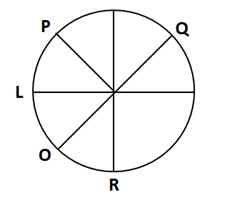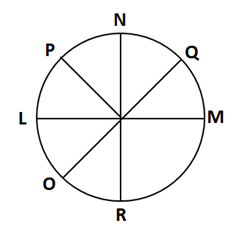### logical reasoning puzzles pdf: logical reasoning puzzles pdf that you should refer to revisit concepts

Logical reasoning puzzles pdf will is the recipe you need during the preparation phase and just before the final exam day. All the important concepts clustered in a single document will reduce panic of searching and referring multiple sources to revise concepts. You can create your own logical reasoning puzzles pdf by studying multiple pds/documents/articles and filtering out the key points to make own notes according to your strengths and weaknesses. Therefore, ensure that you have an organized set of notes to refer before your exam built by absorbing tips from various mock tests solutions.

Directions for the question set:
Study the following information to answer the given questions:
L, M, N, O, P, Q and R are sitting around a circle facing the Centre. O is sitting between L and R. Q is second to the right of R and P is second to the right of Q. N is not an immediate neighbour of R.

Question 1: Which of the following is not correct?
(a) R is second to the right of L
(b) M is second to the left of N
(c) L sits exactly between Q and P
(d) P and N are immediate neighbours
(e) P sits to the opposite of N

Question 2: How many persons are seated between L and Q if we count anticlockwise from L to Q?
(a) One
(b) Two
(c) Three
(d) Four
(e) More than four

Question 3: Who is to the immediate left of P?
(a) L
(b) N
(c) M
(d) O
(e) None of these

Additional Directions (4-5): Four of the following five are alike in a certain way based on their seating positions in the above arrangement and so form a group.

Question 4: Which is the one that does not belong to the group?
(a) QPN
(b) MOR
(c) LRO
(d) RQM
(e) ROL

Question 5: Which of the following is odd in the following?
(a) LP
(b) RM
(c) MQ
(d) NP
(e) OR

### Answers and Explanations: Click the down arrow to expand

Common Solution for the set: Here R Determine the position of Q & L in two statements so, the arrangement will be O is sitting between L and R. Q is second to the right of R and P is second to the right of Q. this means that L is left of R & Q id right of R because both are sitting in same distance and l cannot be right of R because Q is there.N is not an immediate neighbour of R. So, N will Sit between Q & P. M will sit between R & Q Final arrangement will be.Answer 1: (e) P sits by the side of N. The correct option is (e).

Answer 2: (c) For the common solution for this set, refer to solution of Question 1. There are 3 people in the anti-clock wise direction between L and Q. The persons are O, R and M The correct option is (c).

Answer 3: (b) For the common solution for this set, refer to solution of Question 1. N is to the immediate left of P. The Correct option is (b).

Answer 4: (e) For the common solution for this set, refer to solution of Question 1. Unlike all other options ROL are in order in clockwise direction. Also, its 3rd letter is not in the middle of 1st and 2nd. The correct option is (e).

Answer 5: (a) For the common solution for this set, refer to solution of Question 1. The 2nd letter is to the right of the first. And all are in anticlockwise direction but LP is in clockwise direction. Thus, LP is not following the rule. The correct option is (a)

Extra tips for logical reasoning puzzles pdf: